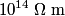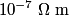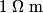## Solution to 1986 Problem 23

 The resistivities of different materials vary over approximately 20 orders of magnitude, with insulators having resistivities on the order of$10^{14} \;\Omega\;\mathrm{m}$, conductors having resistivities on the order of$10^{-7} \;\Omega\;\mathrm{m}$ and semiconductors having resistivities on the order of$1 \;\Omega\;\mathrm{m}$. Therefore, statement (A) is correct. The resistivity of a conductor (like copper) increases with temperature, while the resistivity of a semiconductor (like silicon) decreases with temperature. Therefore, statements (B) and (C) are correct. It is unclear what the effect of adding an impurity to copper will be. So, it is unclear whether or not statement (D) is correct. Doping silicon with impurities such as arsenic or indium is a common method to increase its conductivity. Therefore, statement (E) cannot be correct. Therefore, (E) is the correct answer to the question.